Click to Chat

1800-1023-196

+91-120-4616500

CART 0

• 0

MY CART (5)

Use Coupon: CART20 and get 20% off on all online Study Material

ITEM
DETAILS
MRP
DISCOUNT
FINAL PRICE
Total Price: Rs.

There are no items in this cart.
Continue Shopping• Complete JEE Main/Advanced Course and Test Series
• OFFERED PRICE: Rs. 15,900
• View Details

```Chapter 6: Exponents Exercise – 6.2

Question: 1

Using laws of exponents, simplify and write the answer in exponential form

(i) 23 × 24 × 25

(ii) 512 ÷ 53

(iii) (72)3

(iv) (32)5 ÷ 34

(v) 37 × 27

(vi) (521 ÷ 513) × 57

Solution: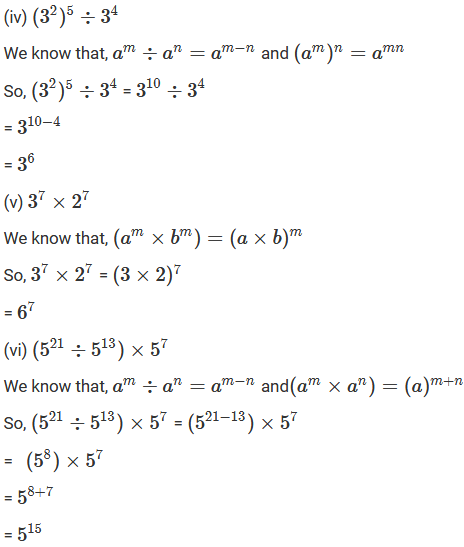Question: 2

Simplify and express each of the following in exponential form

(i) ((23)4 × 28) ÷ 212

(ii) (82 × 84) ÷ 83

(iii) (57/52) × 53Solution: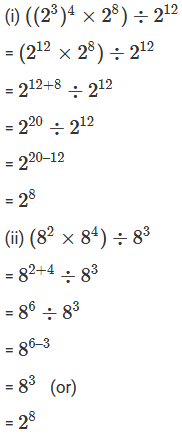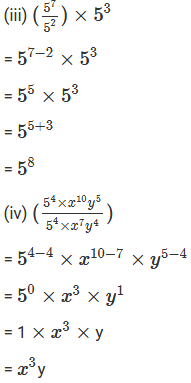Question: 3

Simplify and express each of the following in exponential formSolution: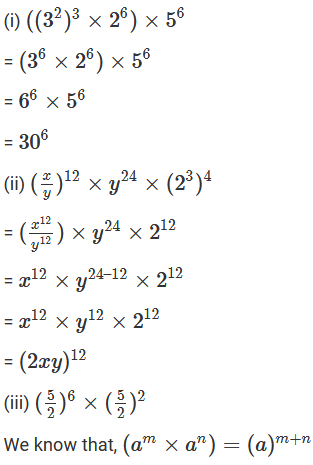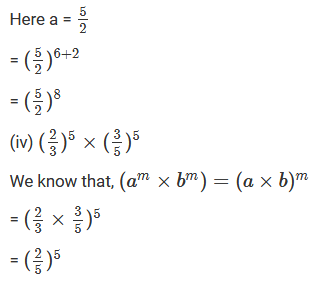Question: 4

Write 9 × 9 × 9 × 9 × 9 in exponential form with base 3

Solution:

9 × 9 × 9 × 9 × 9 = (9)5 = (32)5

= 310

Question: 5

Simplify and write each of the following in exponential form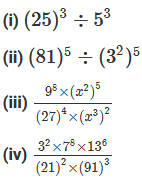Solution: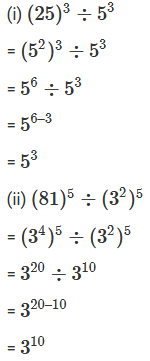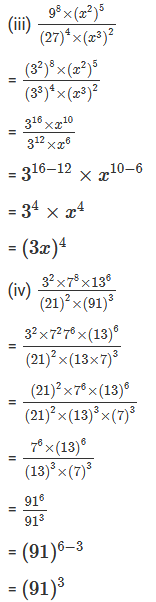Question: 6

Simplify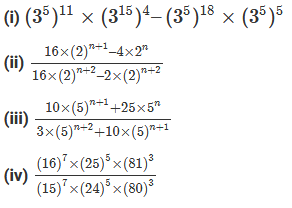Solution: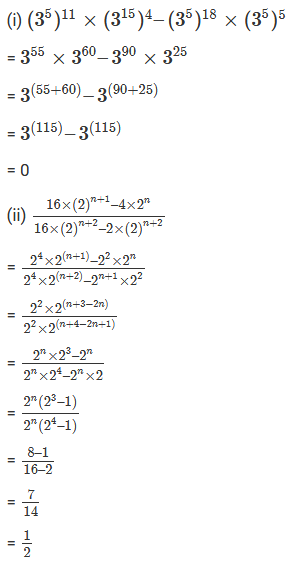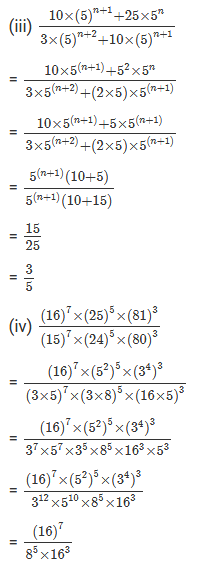Question: 7

Find the values of n in each of the following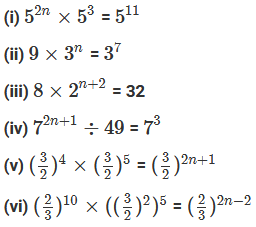Solution: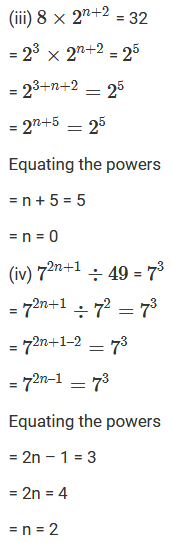Question: 8Solution: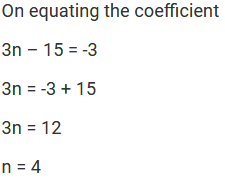```### Course Features

• 728 Video Lectures
• Revision Notes
• Previous Year Papers
• Mind Map
• Study Planner
• NCERT Solutions
• Discussion Forum
• Test paper with Video Solution